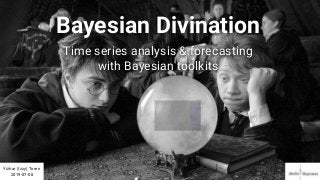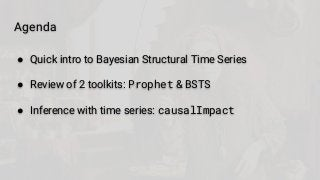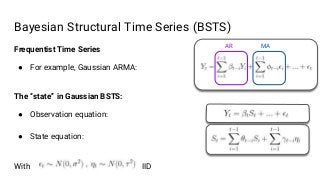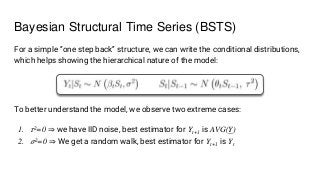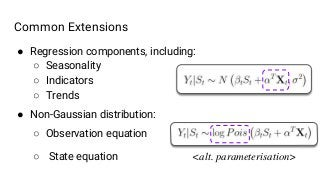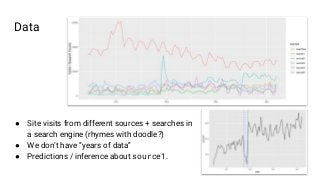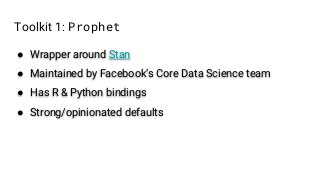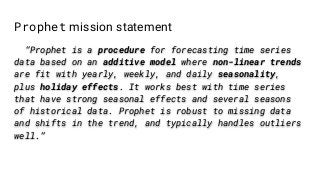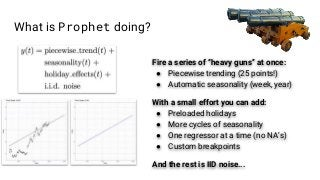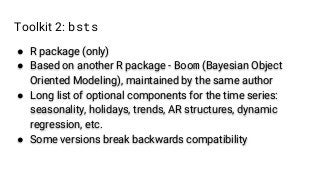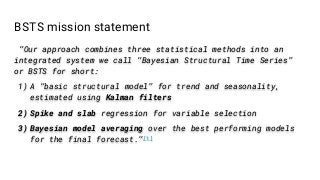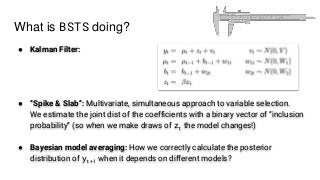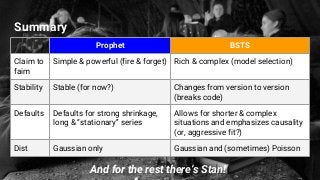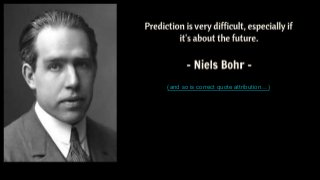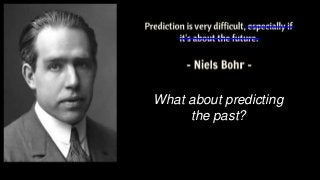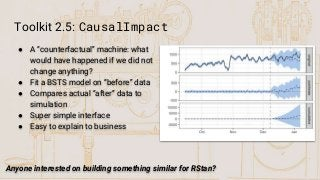1 of 18

### Bayesian Divination: time series analysis & forecasting with Bayesian Toolkits (2019)

1. Bayesian Divination Time series analysis & forecasting with Bayesian toolkits Yizhar (Izzy) Toren 2019-07-08
2. Agenda ● Quick intro to Bayesian Structural Time Series ● Review of 2 toolkits: Prophet & BSTS ● Inference with time series: causalImpact
3. Bayesian Structural Time Series (BSTS) Frequentist Time Series ● For example, Gaussian ARMA: The “state” in Gaussian BSTS: ● Observation equation: ● State equation: With IID AR MA
4. To better understand the model, we observe two extreme cases: 1. 𝜏2=0 ⇒ we have IID noise, best estimator for Yt+1 is AVG(Y) 2. 𝜎2=0 ⇒ We get a random walk, best estimator for Yt+1 is Yt Bayesian Structural Time Series (BSTS) For a simple “one step back” structure, we can write the conditional distributions, which helps showing the hierarchical nature of the model:
5. ● Regression components, including: ○ Seasonality ○ Indicators ○ Trends ● Non-Gaussian distribution: ○ Observation equation ○ State equation Common Extensions <alt. parameterisation>
6. Data ● Site visits from different sources + searches in a search engine (rhymes with doodle?) ● We don’t have “years of data” ● Predictions / inference about source1.
7. Toolkit 1: Prophet ● Wrapper around Stan ● Maintained by Facebook’s Core Data Science team ● Has R & Python bindings ● Strong/opinionated defaults
8. Prophet mission statement “Prophet is a procedure for forecasting time series data based on an additive model where non-linear trends are fit with yearly, weekly, and daily seasonality, plus holiday effects. It works best with time series that have strong seasonal effects and several seasons of historical data. Prophet is robust to missing data and shifts in the trend, and typically handles outliers well.”
9. What is Prophet doing? Fire a series of “heavy guns” at once: ● Piecewise trending (25 points!) ● Automatic seasonality (week, year) With a small effort you can add: ● Preloaded holidays ● More cycles of seasonality ● One regressor at a time (no NA’s) ● Custom breakpoints And the rest is IID noise...
10. Prophet Demo
11. Toolkit 2: bsts ● R package (only) ● Based on another R package - Boom (Bayesian Object Oriented Modeling), maintained by the same author ● Long list of optional components for the time series: seasonality, holidays, trends, AR structures, dynamic regression, etc. ● Some versions break backwards compatibility
12. BSTS mission statement “Our approach combines three statistical methods into an integrated system we call “Bayesian Structural Time Series” or BSTS for short: 1) A “basic structural model” for trend and seasonality, estimated using Kalman filters 2) Spike and slab regression for variable selection 3) Bayesian model averaging over the best performing models for the final forecast.”
13. What is BSTS doing? ● Kalman Filter: ● “Spike & Slab”: Multivariate, simultaneous approach to variable selection. We estimate the joint dist of the coefficients with a binary vector of “inclusion probability” (so when we make draws of zt the model changes!) ● Bayesian model averaging: How we correctly calculate the posterior distribution of yt+1 when it depends on different models?
14. BSTS Demo
15. Summary And for the rest there’s Stan! Prophet BSTS Claim to faim Simple & powerful (fire & forget) Rich & complex (model selection) Stability Stable (for now?) Changes from version to version (breaks code) Defaults Defaults for strong shrinkage, long & “stationary” series Allows for shorter & complex situations and emphasizes causality (or, aggressive fit?) Dist Gaussian only Gaussian and (sometimes) Poisson
16. (and so is correct quote attribution…)
17. What about predicting the past?
18. Toolkit 2.5: CausalImpact ● A “counterfactual” machine: what would have happened if we did not change anything? ● Fit a BSTS model on “before” data ● Compares actual “after” data to simulation ● Super simple interface ● Easy to explain to business Anyone interested on building something similar for RStan?

### Editor's Notes

1. Equations by: https://www.codecogs.com/latex/eqneditor.php
2. Notation from: http://www.unofficialgoogledatascience.com/2017/07/fitting-bayesian-structural-time-series.html LaTeX code: Frequentist: Y_{t} = \sum_{i=1}^{t-1} \beta_{t-i} Y_i + \sum_{i=1}^{t-1} \phi_{t-i} \epsilon_i + ... + \epsilon_t Obs: Y_{t} = \beta_t S_t + ... + \epsilon_t State: S_t = \sum_{i=1}^{t-1} \theta_{t-i} S_i + \sum_{i=1}^{t-1} \gamma_{t-i} \eta_i Variance: \epsilon_t \sim N(0, \sigma^2) \: , \: \eta_t \sim N(0, \tau^2)
3. Latex Code Y_{t} | S_{t} \sim N \left(\beta_t S_t , \sigma^2 \right) S_{t} | S_{t-1} \sim N \left( \theta_{t} S_{t-1} , \: \tau^2 \right)
4. LaTeX code Regression: Y_{t} | S_{t} \sim N \left(\beta_t S_t + \alpha^T \bold{X}_t, \sigma^2 \right)